# Circle Squares fractal

Written by Paul Bourke
January 1990, updated December 2006

This Moire like pattern is created by the function

z2 modulo m

Where z (complex) are points on a rectangular region of the complex plane corresponding to the image being created. M is an integer that determines the colour resolution, typically around 200. modulo arithmetic on a complex number is applied to the magnitude, so

z modulo m = (|z| modulo m) * ( zreal + i zimag) / |z|

Where |z| represents the magnitude of the complex number and is a real number, the modulo of a real number with m is the remainder on division by m. For each z the corresponding position on the image plane is shaded in proportion to the magnitude of z, namely |z|.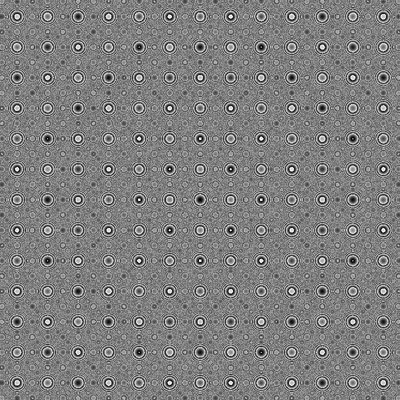-256 < zreal zimag < 256-128 < zreal zimag < 128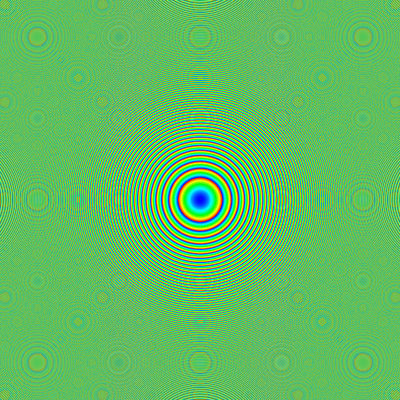Contribution by Tim Meehan

I was doing some research that involved numerical Fresnel diffraction and noticed that one of my images looked very similar to your circle fractals. The function was exp(kj(x2+y2)). The 'k' is a constant, and the function represents the thin lens amplitude transmittance function for Fresnel diffraction. If you take the argument of the previous function, you get images that look very similar to your circle squares.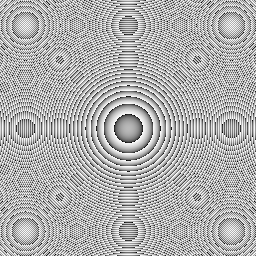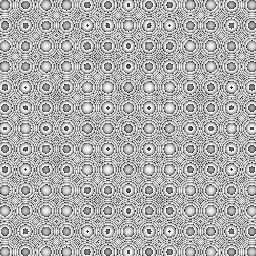Also, if you take the real (or imaginary) part the variation from dark to light is a lot smoother and nicer.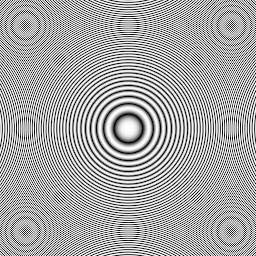Python code: thin_lens.py and bmp.py.

Contribution by Santiago Zubieta

Santiago has found similar patterns while exploring bit-wise and modulus operations on raw pixel indices. Java code fragment follows:

```for (int i=0; i<width; ++i) {
for (int j=0; j<height; ++j) {
int x = i*i-2*(i|j)+j*j;
x %= 255;
x = Math.abs(x);
g.setColor(new Color(x,x,x));
g.drawRect(i, j, 1, 1);
}
}```Directional grep scale ramp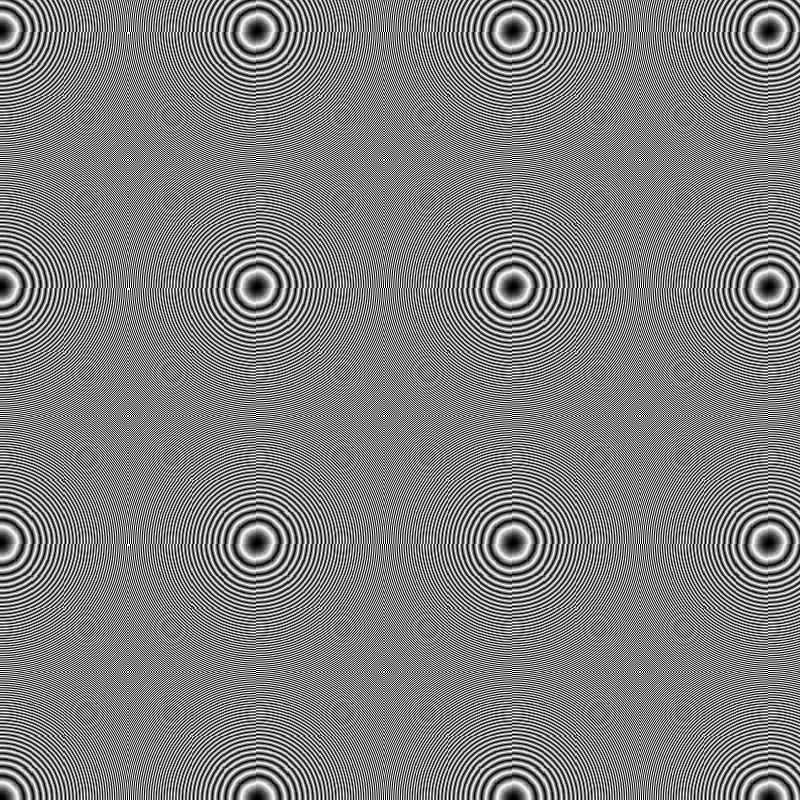Periodic grep scale ramp